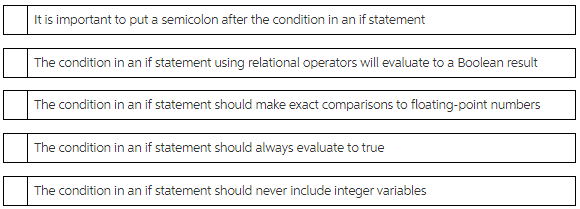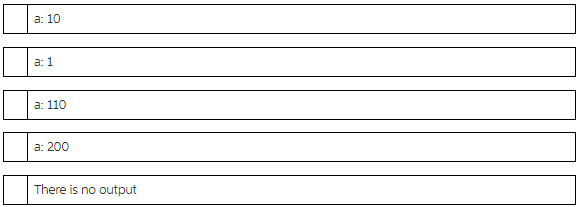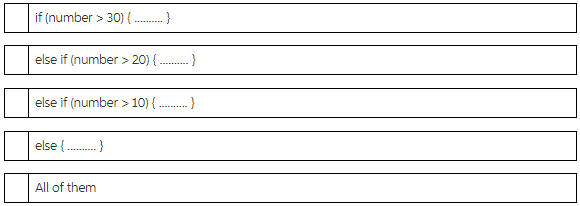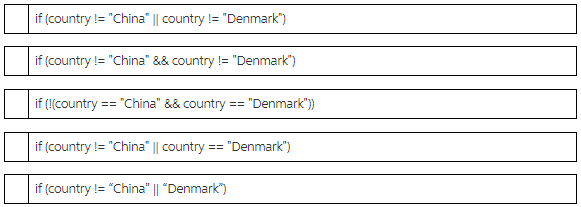# Which statement about an if statement is true?

Which statement about an if statement is true?Flag this Question

Question 2 2.5 pts

Assuming that the user provides 100 as input, what is the output of the following code snippet?

int a =10, b;
cout << "Please enter b: ";
cin >> b;
if (b > 100)
a = b;
a = a + b;
cout << "a: " << a << endl;Flag this Question

Question 3 2.5 pts

Consider the following code snippet:

int number = 0;
cout << "Enter number: ";
cin >> number;
if (number > 30)
{ … }
else if (number > 20)
{ … }
else if (number > 10)
{ … }
else { … }

Assuming that the user input is 40, which of the following block of statements is executed?Flag this Question

Question 4 2.5 pts

Which of the following options checks that country is neither China nor Denmark?